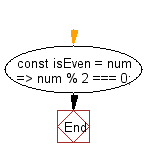# JavaScript: Return true if the given number is even, false otherwise

## JavaScript fundamental (ES6 Syntax): Exercise-199 with Solution

Write a JavaScript program that returns true if the given number is even, false otherwise.

• Checks whether a number is odd or even using the modulo (%) operator.
• Returns true if the number is even, false if the number is odd.

Sample Solution:

JavaScript Code:

``````//#Source https://bit.ly/2neWfJ2
const isEven = num => num % 2 === 0;

console.log(isEven(3));
console.log(isEven(32));
console.log(isEven(1));
console.log(isEven(0));
```
```

Sample Output:

```false
true
false
true
```

Pictorial Presentation:Flowchart:Live Demo:

See the Pen javascript-basic-exercise-199-1 by w3resource (@w3resource) on CodePen.

Improve this sample solution and post your code through Disqus

What is the difficulty level of this exercise?

Test your Programming skills with w3resource's quiz.

﻿